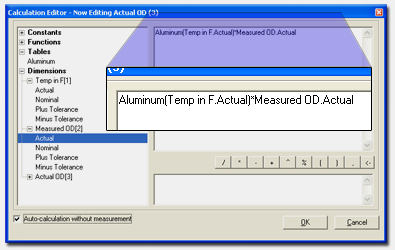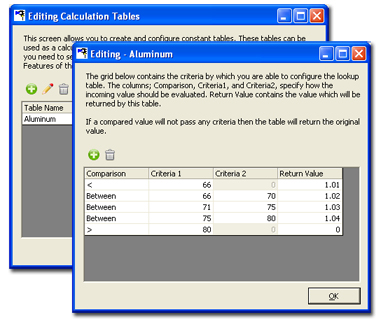Calculated Dimensions
Calculated Dimensions
QC-Gage allows you to create dimensions that are based on calculations. These can either be based on an entered value or on the values of other dimensions.

Calculations without Values
Checking the "Auto-calculation without measurement" checkbox will cause QC-Gage to automatically calculate the value of the feature without your entry of an actual measurement value. The example on the left shows a feature called Actual OD that is being calculated based on the result of the lookup of Temperature multiplied by the Measured OD. There is no need to enter a value for Actual OD since it will auto-calculate.

Calculations with Values
Also optional is the ability to calculate a new value based on the value received for the measurement. This is similar to the Actual Offset feature in QC-Gage 3.0 that allowed you to add an offset value to the actual measured value that came from the gage.

Lookup Tables
In the Calculated Dimensions, you can create shared lookup tables. These lookup tables can be queried as a part of the calculation in order to obtain constant values. In the example on the right, the temperature is passed into the Aluminum lookup table. The returned value from the lookup table is then multiplied by the measured value to calculate the adjusted actual. This feature allows you to account for situations like thermal compensation.